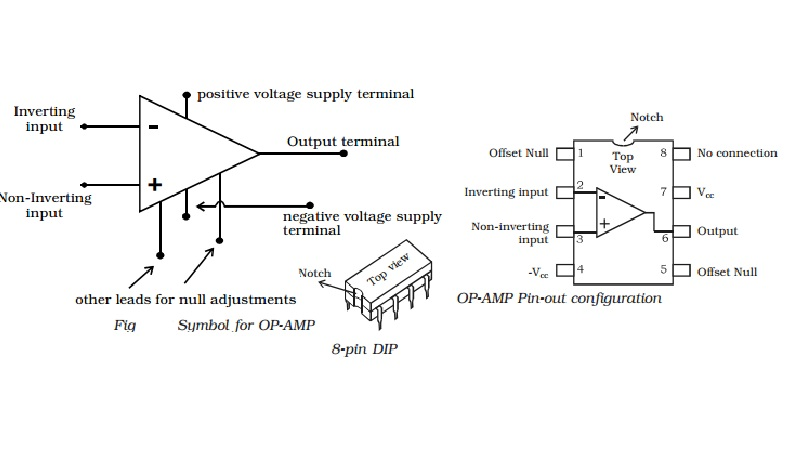Home | | Physics | Circuit symbol and Pin-out configuration of an OP-AMP

# Circuit symbol and Pin-out configuration of an OP-AMPThe OP - AMP is represented by a triangular symbol as shown in Fig. It has two input terminals and one output terminal.

Operational amplifier (OP - AMP)

Linear integrated circuits are being used in a number of electronic applications, such as in the fields like communication, medical electronics, instrumentation control etc. An important linear IC is an operational amplifier.

OP-AMP is a solid state device capable of sensing and amplifying dc and ac input signals. OP-AMP is an amplifier with two inputs (differential inputs) and a single output. OP-AMP consists of 20 transistors, 11 resistors and one capacitor. It usually requires a positive and negative power supply (dual power supply). This allows the output voltage to swing positive and negative with respect to ground.

The most important characteristics of OP-AMP are : (i) very high input impedance or even infinity which produces negligible current at the inputs, (ii) very high gain, (iii) very low output impedance or even zero, so as not to affect the output of the amplifier by loading.

An OP-AMP is so named, because it was originally designed to perform mathematical operations such as addition, subtraction, multiplication, division, integration, differentiation etc in analog computer. Nowdays OP-AMPs are used in analog computer operations and in timing circuits.

Circuit symbol and Pin-out configuration of an OP-AMP

The OP - AMP is represented by a triangular symbol as shown in Fig. It has two input terminals and one output terminal. The terminal with negative sign is called as the inverting input and the terminal with positive sign is called as the non-inverting input. The input terminals are at the base of the triangle. The output terminal is shown at the apex of the triangle.The widely used very popular type Op-Amp IC 741, which is available in DIP. Referring to the top view of the dual-in-package, the pin configuration of IC 741 can be described (Fig) as follows. The top pin on the left side of the notch indicates Pin 1. The pin number 2 is inverting input terminal and 3 is non-inverting input terminal. Pin 6 is the output terminal. A d.c. voltage or a.c signal placed on the inverting input will be 180o out of phase at the output. A d.c. voltage or a.c. signal placed on the non-inverting input will be inphase at the output. Pins 7 and 4 are the power supply terminals. Terminals 1 and 5 are used for null adjustment. Null adjustment pins are used to null the output voltage when equal voltages are applied to the input terminals for perfect balance. Pin 8 indicates no connection.Study Material, Lecturing Notes, Assignment, Reference, Wiki description explanation, brief detail
11th 12th std standard Class Physics sciense Higher secondary school College Notes : Circuit symbol and Pin-out configuration of an OP-AMP |